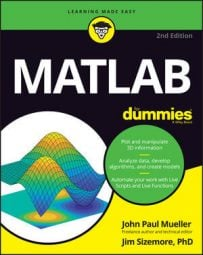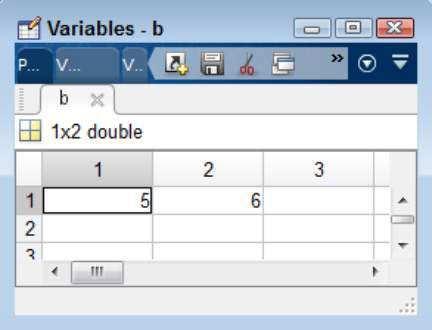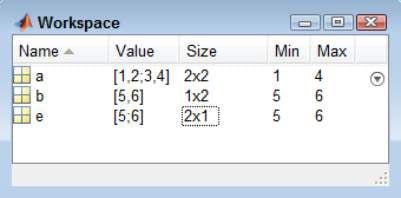##### MATLAB For DummiesThink about how you use data when working with math: The data appears as a list of numbers or text. MATLAB uses a similar viewpoint. It also works with lists of numbers and text that you create through various methods.

## Entering values inside square brackets

The left square bracket, [, starts a list of numbers or text. The right square bracket, ], ends a list. Each entry in a list is separated by a comma (,). To try this technique yourself, open MATLAB, type b=[5, 6] in the Command window, and press Enter. You see

```b =
5  6```

The information is stored as a list of two numbers. Each number is treated as a separate value. Double-click b in the Workspace window and you see two separate entries. Notice that the Workspace window shows b as a 1 x 2 list in which the entries flow horizontally.You can type format compact and press Enter to save display space. If you want to clear space in the Command window for typing additional commands, type clc and press Enter.

## Starting a new line or row with the semicolon

The comma creates separate entries in the same row. You use the semicolon (;) to produce new rows. To try this technique yourself, type e=[5; 6] in the Command window and press Enter. You see

```e =
5
6```

The information is stored as a list of two numbers. However, the arrangement of the numbers differs. Double-click e in the Workspace window and you see two separate entries. Notice that the Workspace window shows e as a 2 x 1 list in which the entries flow vertically.## Separating values with a comma or a semicolon

It’s possible to create a matrix by combining commas and semicolons. The commas separate entries in the same row and the semicolons create new rows. To see this for yourself, type a=[1, 2; 3, 4] in the Command window and press Enter. You see

```a =
1  2
3  4```

## Finding dimensions of matrices with the Size column

There’s an easier method for obtaining the size of a numeric list easier method. Right-click the Workspace window column list and select Size from the context menu.

You may also find it helpful to display the minimum and maximum values for each entry. This information comes in handy when working with large vectors or matrices where the minimum and maximum values aren’t obvious. To obtain this information, choose Columns→Min, and then choose Columns→Max.## Creating a range of values using a colon

Typing each value in a list manually would be time-consuming and error-prone because you’d eventually get bored doing it. Fortunately, you can use the colon (:) to enter ranges of numbers in MATLAB. The number on the left side of the colon specifies the start of the range, and the number on the right side of the colon specifies the end of the range.

To see this for yourself, type g=[5:10] and press Enter. You see

```g =
5  6  7  8  9 10```

## Creating a range of values using linspace()

Using the colon to create ranges has a problem. MATLAB assumes that the step is 1. However, you may want the numbers separated by some other value. For example, you might want to see 11 values between the range of 5 and 10, instead of just 6.

The linspace() function solves this problem. You supply the starting value, the ending value, and the number of values you want to see between the starting and ending value. To see how linspace() works, type g=linspace(5,10,11) and press Enter. You see

```g =
Columns 1 through 5
5.0000 5.5000 6.0000 6.5000 7.0000
Columns 6 through 10
7.5000 8.0000 8.5000 9.0000 9.5000
Column 11
10.0000```

In this case, the step value is 0.5. Each number is 0.5 higher than the last, and there are 11 values in the output. The range is from 5 to 10. In short, using linspace() is a little more flexible than using the colon, but using the colon requires less typing and is easier to remember.

## Adding a step to the colon method

It turns out that you can also specify the step when using the colon method. However, in this case, you add the step between the beginning and ending of the range when defining the range. So, you type the beginning number, the step, and the ending number, all separated by colons. To try this method for yourself, type g=[5:0.5:10] and press Enter. You see

```g =
Columns 1 through 5
5.0000 5.5000 6.0000 6.5000 7.0000
Columns 6 through 10
7.5000 8.0000 8.5000 9.0000 9.5000
Column 11
10.0000```

This is precisely the same output as that of the linspace() example. However, when using this method, you specify the step directly, so you don’t control the number of values you receive as output. When using the linspace() approach, you specify the number of values you receive as output, but MATLAB computes the step value for you.

## Transposing matrices with an apostrophe

Using the colon creates row vectors. However, sometimes you need a column vector instead. To create a column vector, you end the input with an apostrophe. To see how this works for yourself, type h=[5:0.5:10]' and press Enter. You see

```h =
5.0000
5.5000
6.0000
6.5000
7.0000
7.5000
8.0000
8.5000
9.0000
9.5000
10.0000```

When you look at the Workspace window, you see that g is a 1 x 11 vector, while h is an 11 x 1 vector. The first entry is a row vector and the second is a column vector.

You can transpose matrices as well. The rows and columns change position. For example, earlier you typed a=[1,2;3,4], which produced

```a =
1  2
3  4```

To see how this matrix looks transposed, type i=[1,2;3,4]' and press Enter. You see

```i =
1  3
2  4```